### Formula E Circuits

•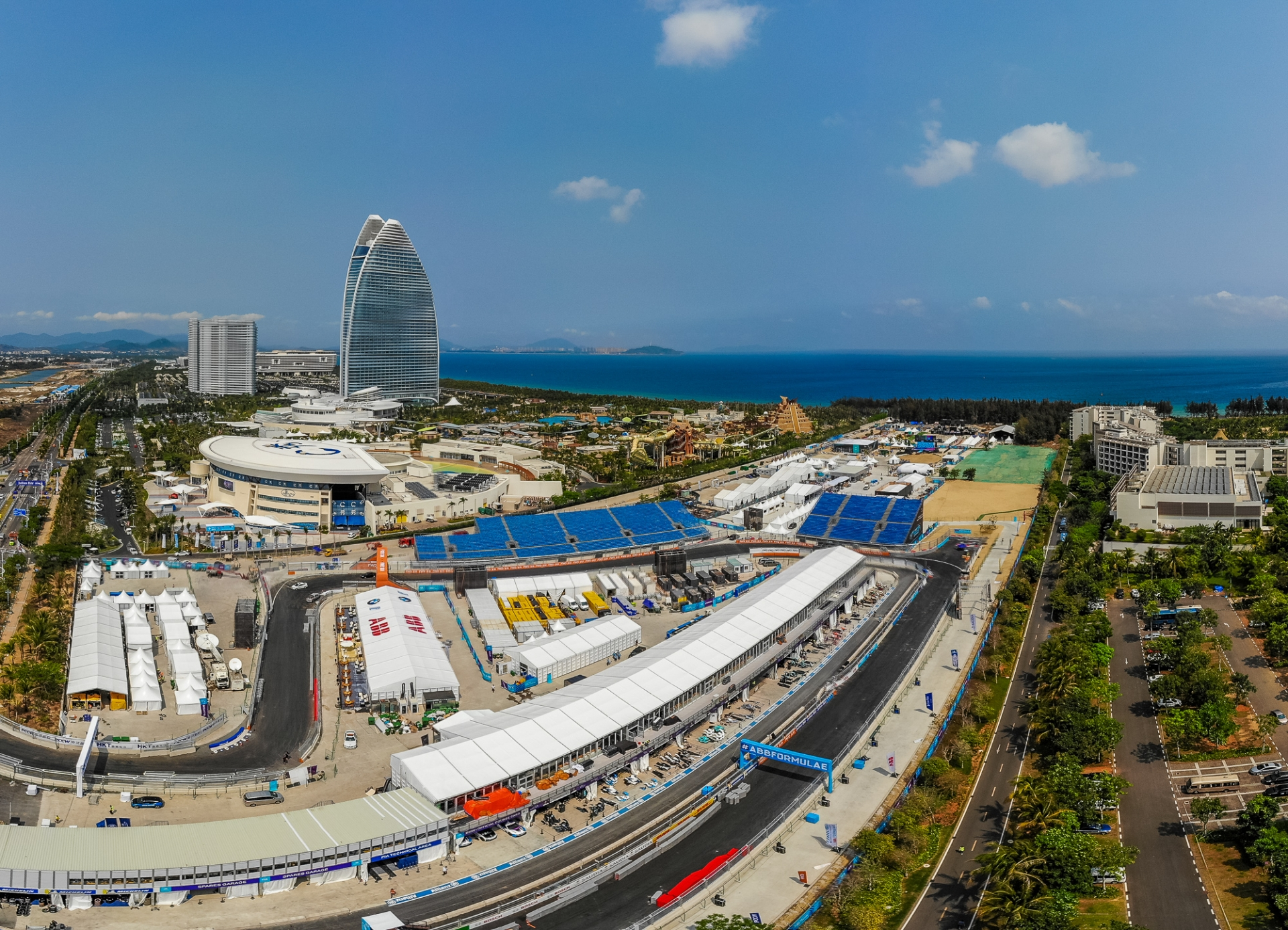Sanya Street Circuit
Formula E
•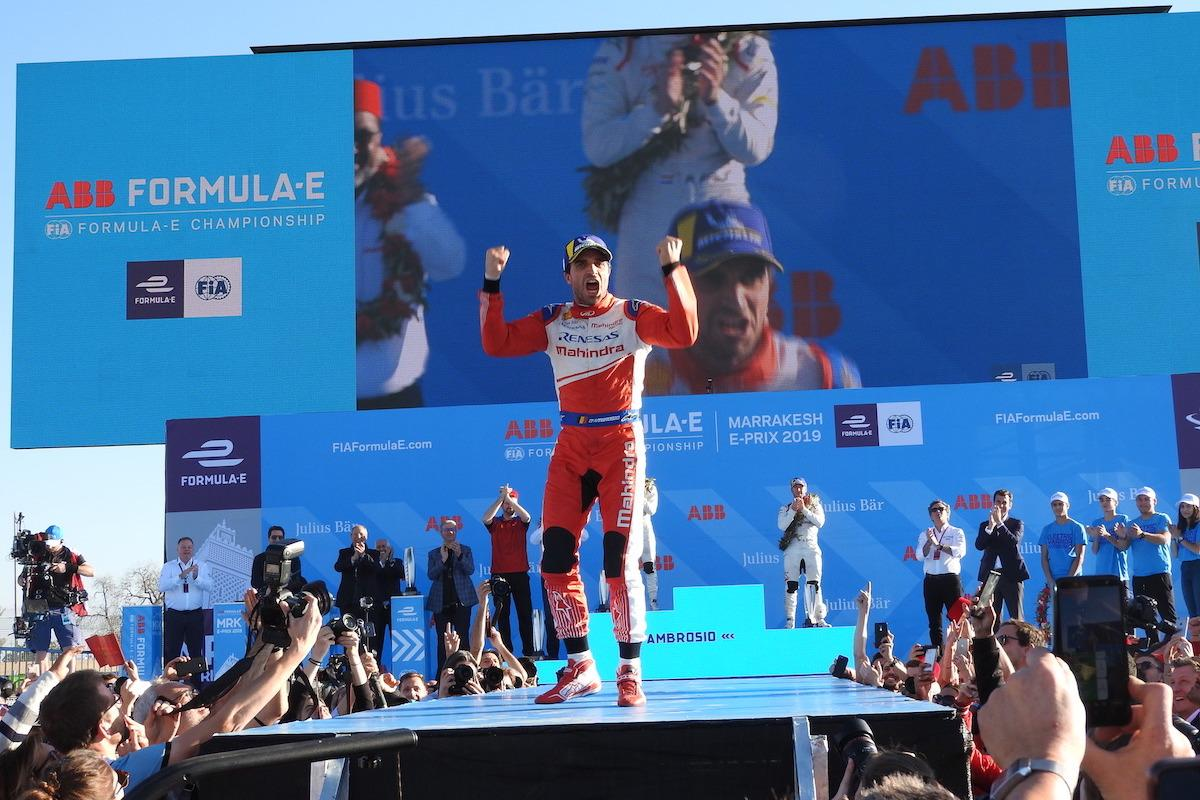Circuit Moulay El Hassan Marrakesh
Formula E, WTCR
•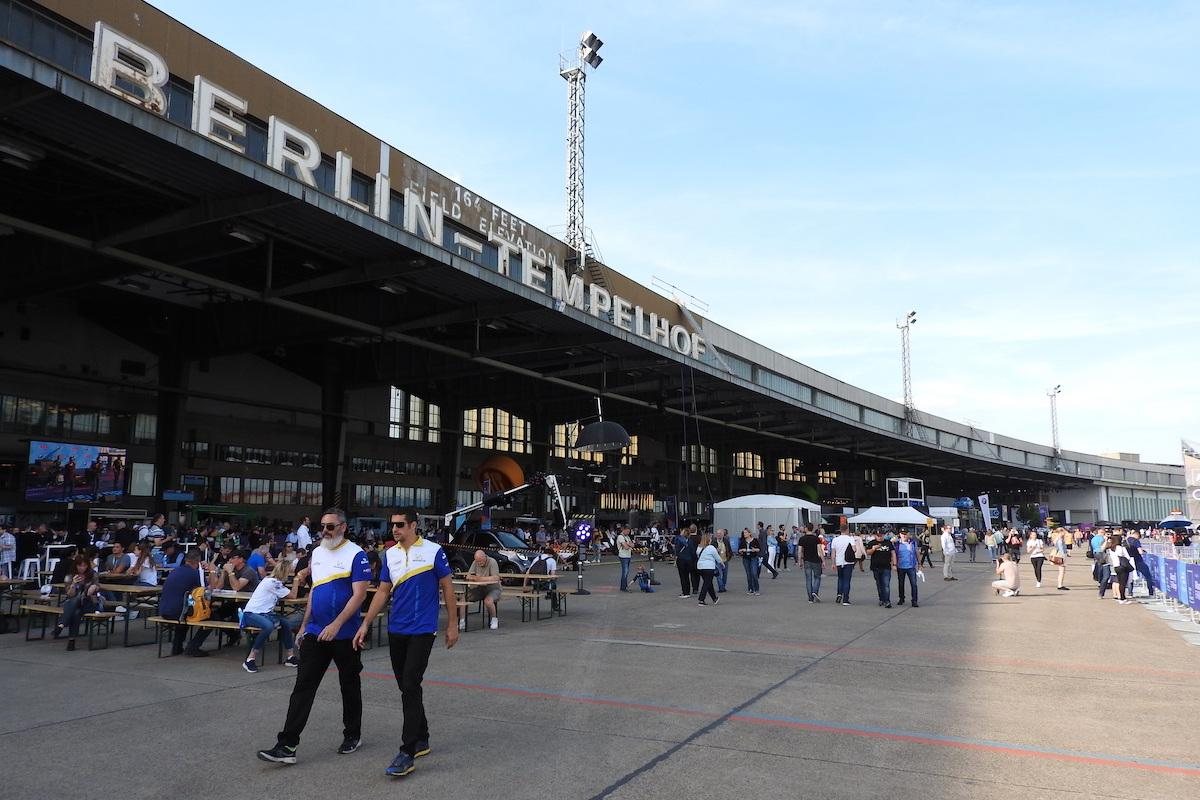Tempelhof Airport Street Circuit
Formula E
•Parque O'Higgins Circuit
Formula E
•Circuit des Invalides - Paris Street Circuit
Formula E
•ExCel London Formula E Circuit
Formula E
•Seoul Street Circuit
Formula E
•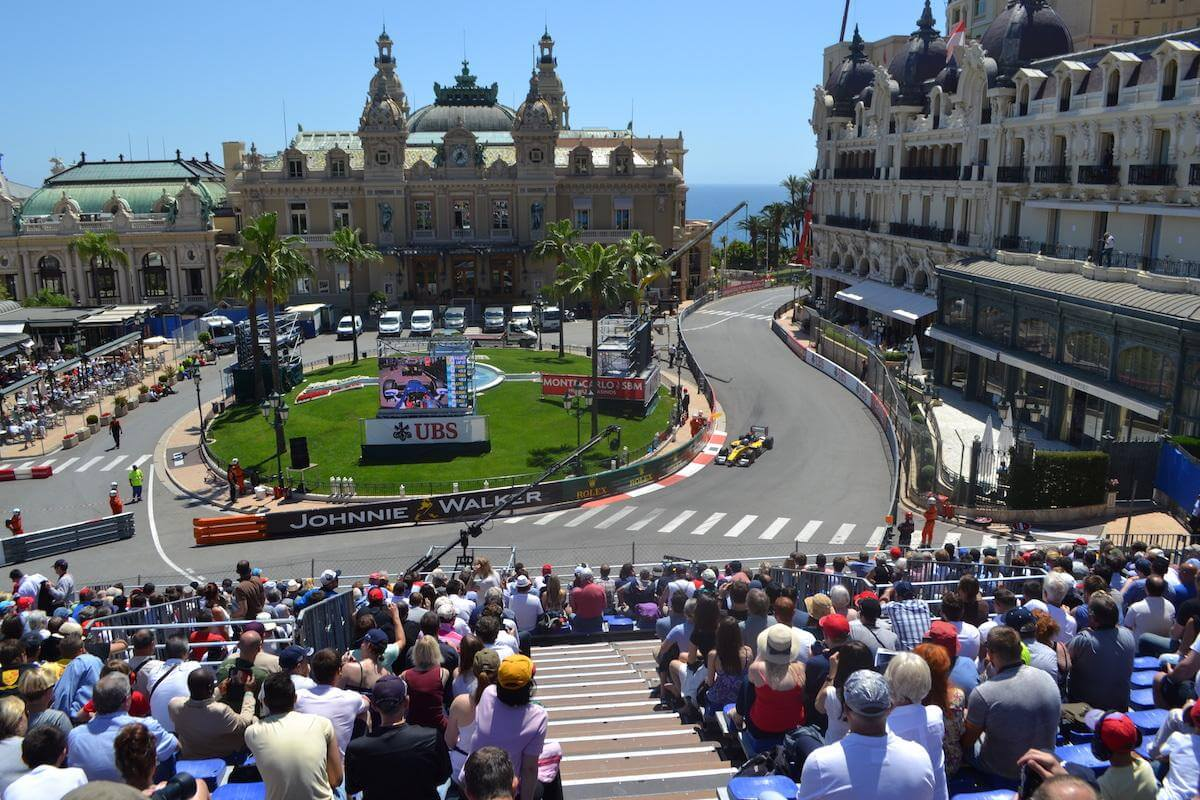Circuit de Monaco
Formula 1, Formula E
•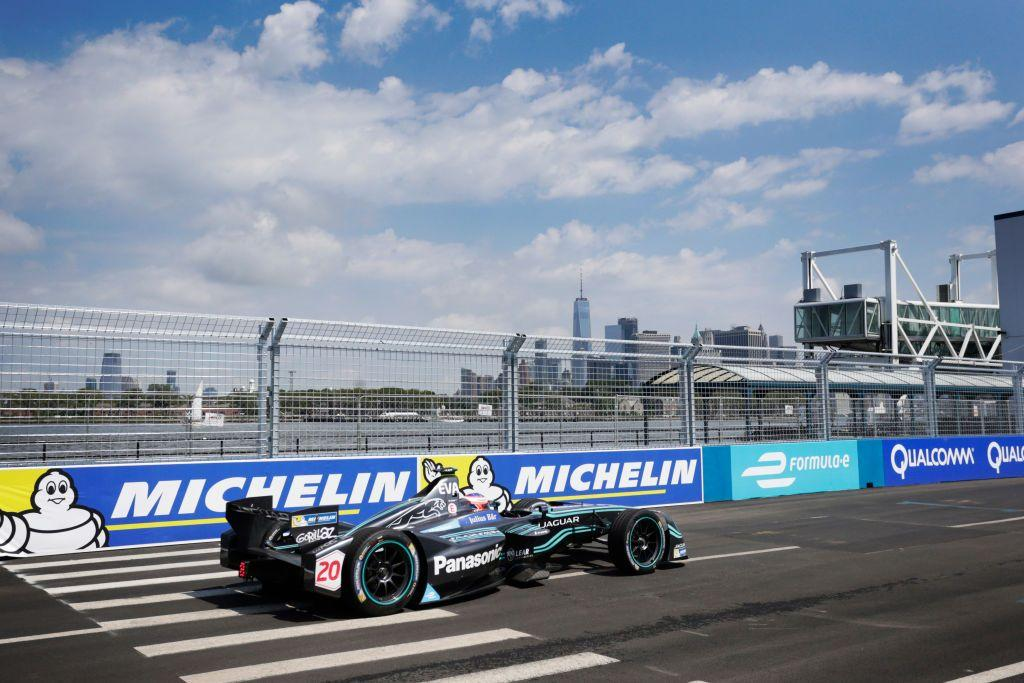Brooklyn Street Circuit
Formula E
•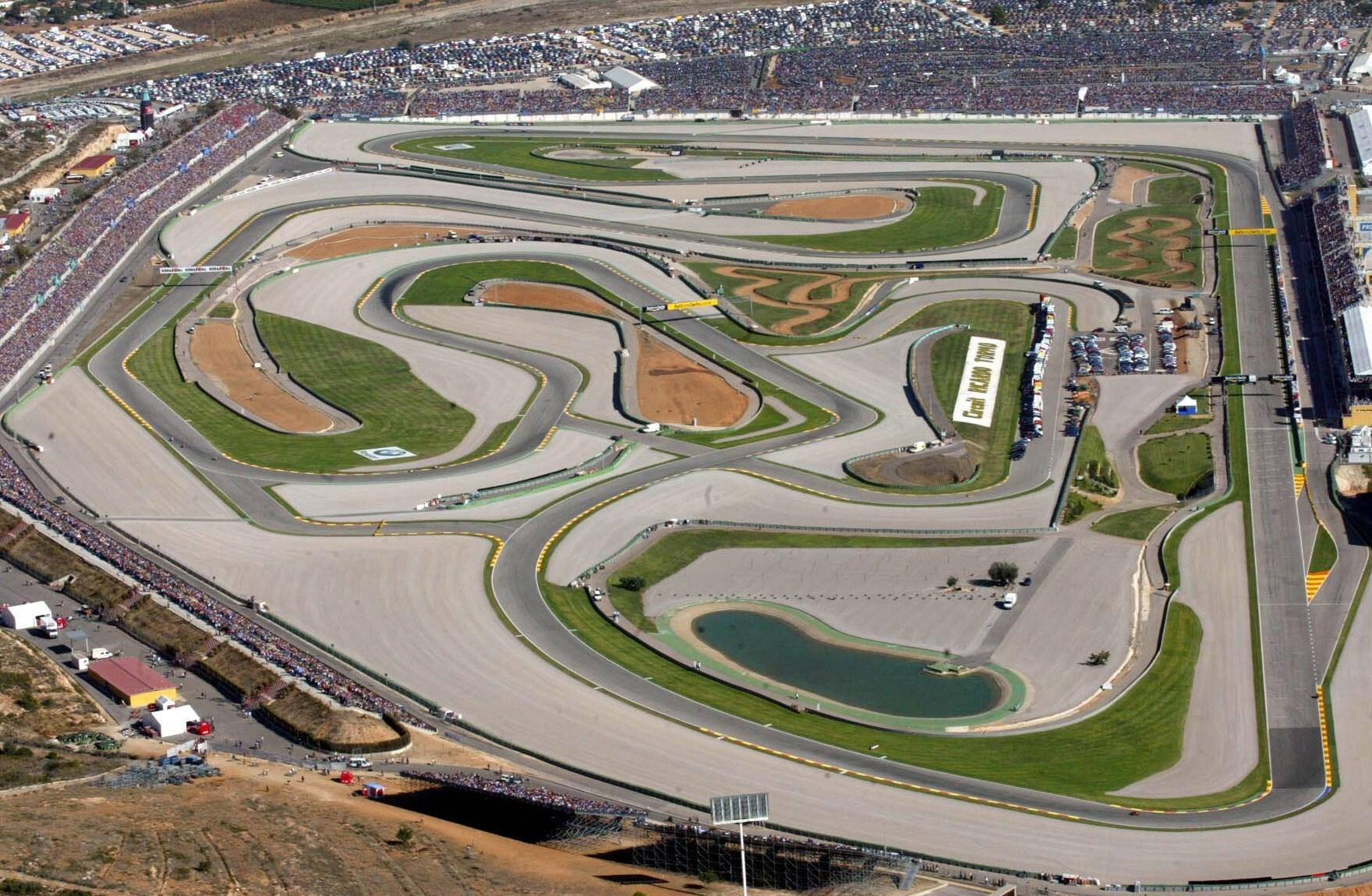Circuit Ricardo Tormo Valencia
MotoGP, Formula E
•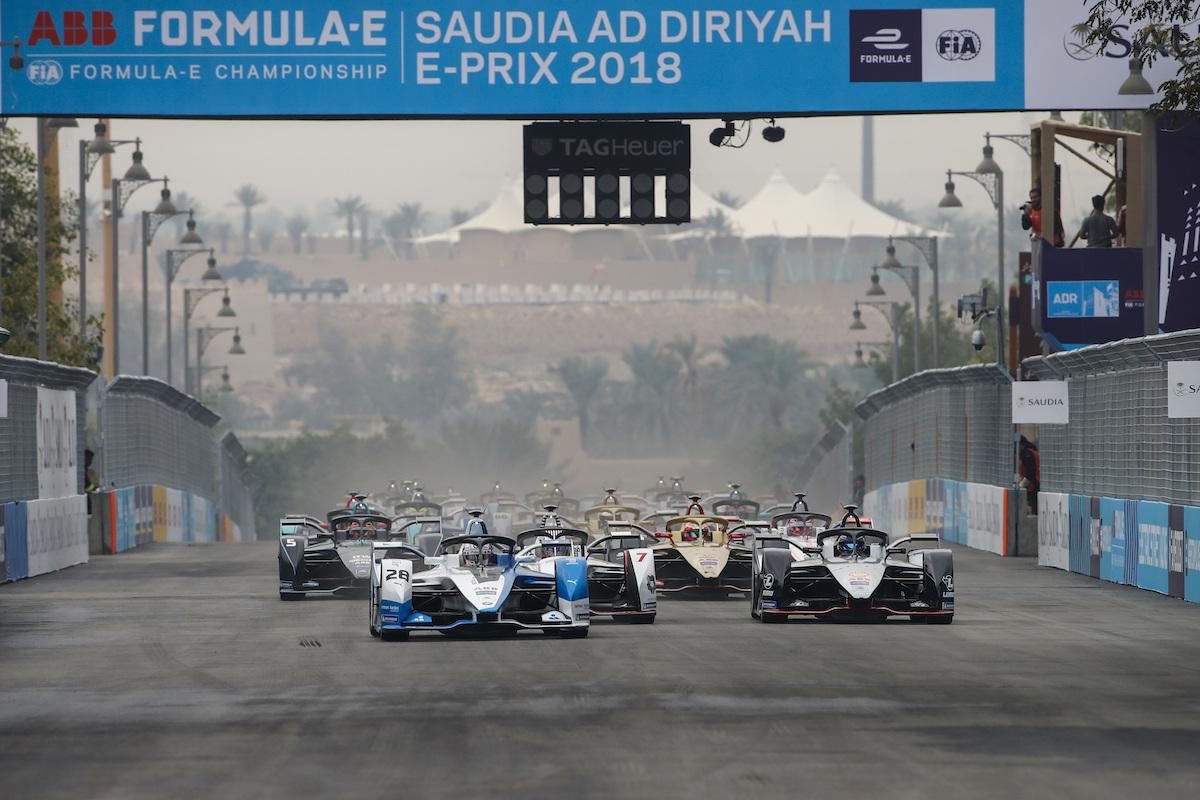Formula E
•Jakarta Street Circuit
Formula E
•Vancouver Street Circuit
Formula E
•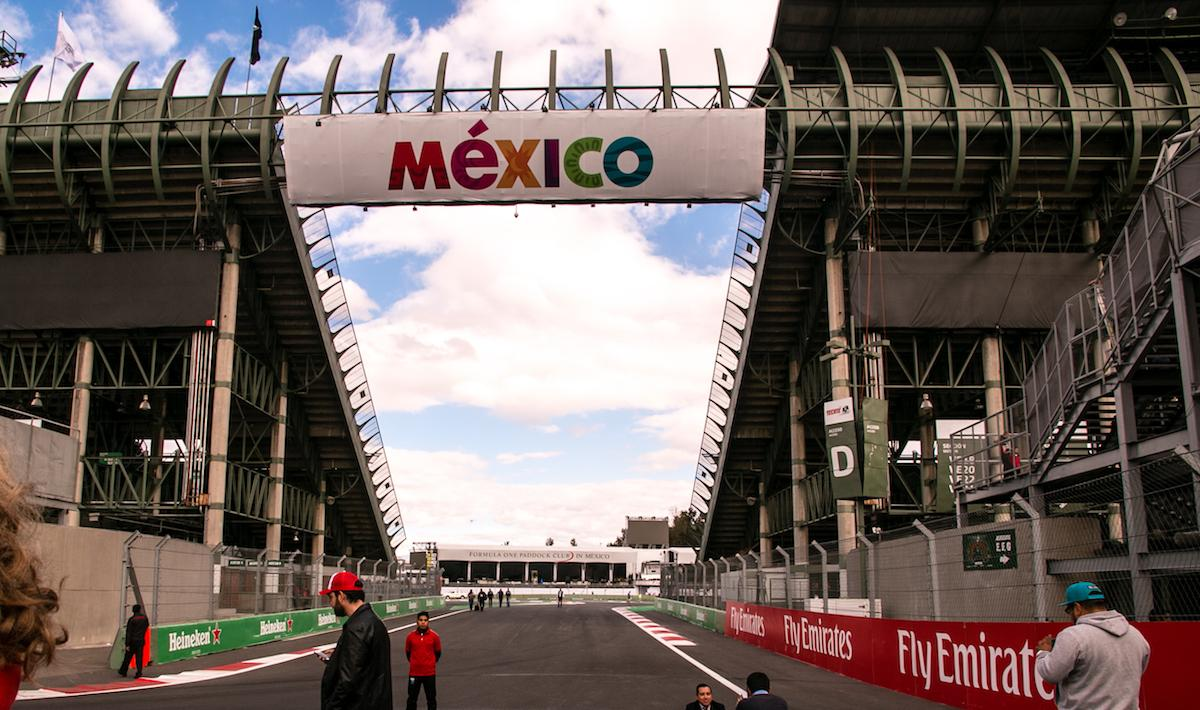Autodromo Hermanos Rodriguez
Formula 1, Formula E
•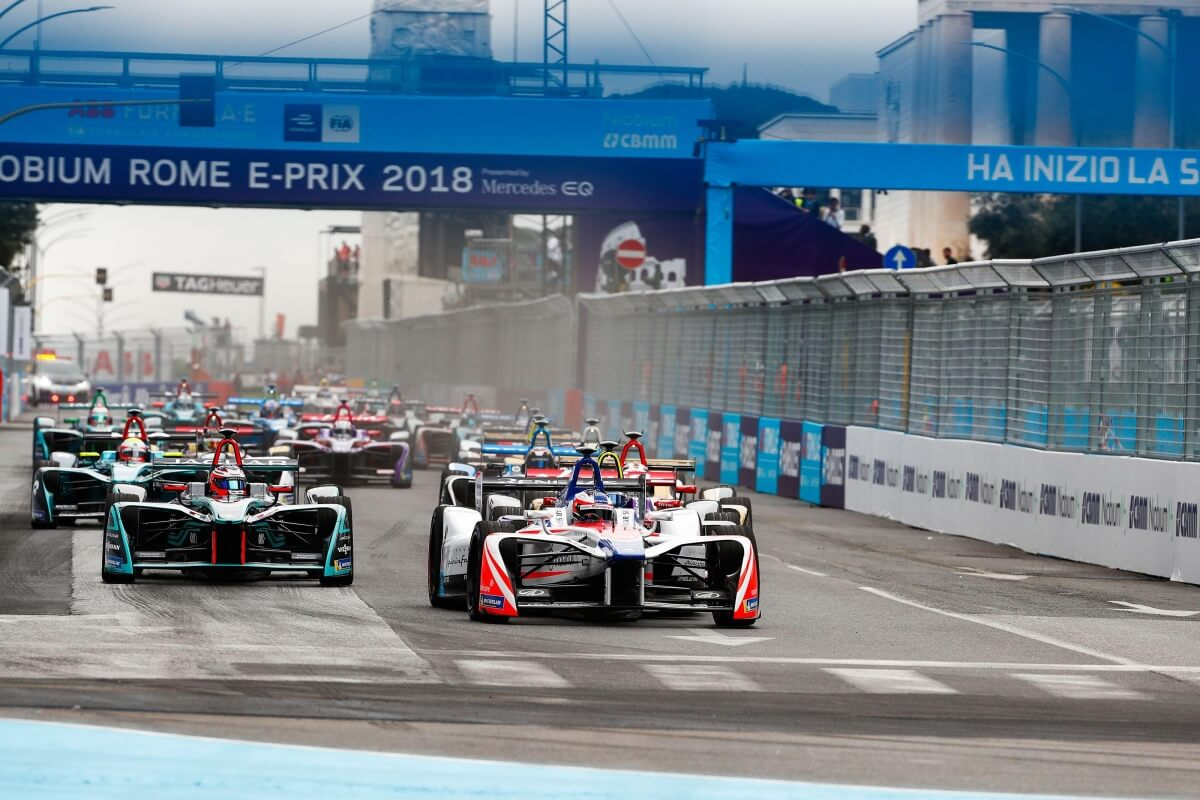Circuito Cittadino dell'EUR - Rome Street Circuit
Formula E

### Formula E Upcoming Events

There are no upcoming events at this time.